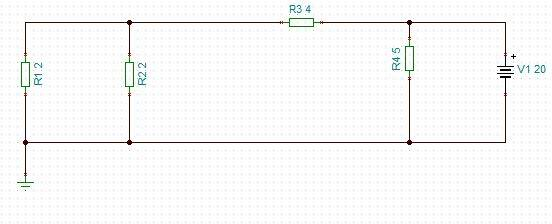# DC current through a resistor circuit

• Tim86
You know that the total current from the source is 8A (congratulations, BTW, that is maybe the hard part of this problem). Now, What is the current through R4? If the current from the voltage source is known and the current through R4 is know what must the current through R3 be?This is where I got the 4.4...A from.If the current through R3 was to be 4.44 amp;then the resistance of the R1,2,3 branch would need to be 20 / 4.44 = 4.505 ohms.So the question is; How could you get ( ( R1 // R2 ) +f

#### Tim86

Thread moved from the technical forums, so no Homework Template is shown.
Summary:: Current through a certain resistor in a mixed dc circuit.

Hi, Sorry if this is the wrong forum.

I'm trying to work out the current through R1.The answer I need is 2 Amp, which I have confirmed with a simulation software.I have calculated the source current as 8A, the current past R3 as 4.44...A and finally R1 as 2.22...A.

Can anyone tell me what I am doing wrong please?

thanks

And how can be anyone able to say what you did wrong, without knowing what you did?

Do these numbers - 2, 3, 4, 5 - mean resistance in ohms? If so, the only number that looks OK is 8A total.

I have calculated the source current as 8A, the current past R3 as 4.44...A and finally R1 as 2.22...A.

Can anyone tell me what I am doing wrong please?
Yes, no, no.

No, we can only guess at what you might have done.

hint: what is the current through R4?

Can anyone tell me what I am doing wrong please?
If the current through R3 was to be 4.44 amp;
then the resistance of the R1,2,3 branch would need to be 20 / 4.44 = 4.505 ohms.

So the question is; How could you get ( ( R1 // R2 ) + R3 ) = 4.505 ohm ?
( Where the double diagonal symbol "//" represents resistors in parallel ).
Maybe you calculated the resistance of the parallel ( R1 // R2 ) as 0.5 ohm, NOT as 1 ohm.
Then, when added to R3 = 4 ohm you get 4.5 ohm which is wrong.

To find the the current through R1.
The current through R4 is irrelevant.
Current through R3 is 20 volt / ( R3 + ( R1 // R2) ) ohm = 20 / 5 = 4 amp.
Since R1 and R2 are equal, half that flows through each; 4 amp / 2 = 2 amp.

If the current through R3 was to be 4.44 amp;
then the resistance of the R1,2,3 branch would need to be 20 / 4.44 = 4.505 ohms.

So the question is; How could you get ( ( R1 // R2 ) + R3 ) = 4.505 ohm ?
( Where the double diagonal symbol "//" represents resistors in parallel ).
Maybe you calculated the resistance of the parallel ( R1 // R2 ) as 0.5 ohm, NOT as 1 ohm.
Then, when added to R3 = 4 ohm you get 4.5 ohm which is wrong.

To find the the current through R1.
The current through R4 is irrelevant.
Current through R3 is 20 volt / ( R3 + ( R1 // R2) ) ohm = 20 / 5 = 4 amp.
Since R1 and R2 are equal, half that flows through each; 4 amp / 2 = 2 amp.

I think my mistake was not ignoring R4, what is the reason for that? Does the current not get divided between branch R3 and R4?

I took the 8 Amps and worked out the current division through R3 (R3/R3+R4*IS(8A))

This is where I got the 4.4...A from.

I think my mistake was not ignoring R4, what is the reason for that? Does the current not get divided between branch R3 and R4?

I took the 8 Amps and worked out the current division through R3 (R3/R3+R4*IS(8A))

This is where I got the 4.4...A from.